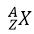Nuclear physics

It has been observed that those elements whose charge number Z is greater than 82 are unstable. Some invisible radiations, that can affect the photographic plates emanate out of these elements. Such elements are called radioactive and the phenomenon is called radioactivity. The radiations coming out of the radioactive elements are called alpha (α), beta (β), and gamma (γ) radiation.

Radioactivity was discovered by Henri Becquerel in 1896. He found that an ore containing uranium (Z=92) emits invisible radiation that penetrates through a black paper wrapping a photographic plate and affects the plate. After becquerel’s discovery, Marie Curie and Pierre Curie discovered two new radioactive elements that they called polonium and radium.

The analysis of the radiations emanating out of radioactive material can be carried out by a simple experiment. The radioactive material is placed at the center of a block of lead by drilling a hole in the block. Radioactive radiations enter a vacuum chamber after emerging out of this hole. After passing between the two parallel plates the radiations strike a photographic plate. These radiations, instead of impinging at one point, fall at three different points due to the potential difference between the plates.

From this experiment, it can be concluded that all radiations from the radioactive material are not alike. The radiation that bends towards the negative plate is made up of positively charged particles. These are called α-particles. Those radiations that bend towards the positive plate are composed of negatively charged particles. These are called β-particles. Those radiations that go straight without bending have no charge. These are called γ-rays.

Further experiments reveal that α-particles are helium nuclei. The charge on them is + Ze while their mass is 4u (atomic mass unit) that is every α-particle has two protons and two neutrons in it. β-particles are in fact fast-moving electrons that come out of the nucleus of a radioactive element. γ-rays like X-rays are electromagnetic waves which issue of the nucleus of a radioactive element. The wavelength of these rays is much compared with the wavelength of X-rays.

### Nucleus Transmutation:

Radioactive is purely a nucleus phenomenon. This is not affected /radiation is emitted out of any radioactive element, it is always accompanied by some changes in the nucleus of the element. Therefore, this element changes into a new element. This phenomenon is called radioactive decay. The element formed due to this change is called the daughter element. The original element is called the parent element. During the nuclear changes, the laws of conservation of mass, energy, momentum, and charge remain applicable.

We knew that three types of radiations α-particle and γ-rays are emitted by the naturally occurring radioactive elements. When α-particle is emitted out of any nucleus then due to the law of conservation of matter the mass number of the nucleus decreases by 4, and due to the law of conservation of charge, the charge of the nucleus decreases by a magnitude of 2e i.e., the charge number of the nucleus decreases by 2. It is due to the fact that the mas number and charge number of emitted particle α are 4 and 2 respectively. The emission of the αparticle is represented by the following equation:Here X represents the parent and Y the daughter element.

To explain the emission of α-particles we take the example of radium. The emission of α-particle from radium 226, results in the formation of radon gas. This change is represented by the following equation.It may be remembered that the sum of the mass numbers and the charge numbers on both sides of the equation are equal. When a β-particle is emitted out of any nucleus, then its mass number does not undergo any change but its charge number is increasing by one. The emission of a β-particle from any element X is represented by the following equation:Negative β-particle is an electron and its emission from the nucleus becomes an incomprehensible enigma, as there is no electron from the nucleus that can be thought of like a neutron emitting an electron and becoming a proton, although the modern explanation is not that simple.

This means that the β-particle is formed at the time of emission. That is why at the time of emission of a β-particle the charge number of the nucleus increases by one but no change in its mass number takes place at the mass of a proton or a neutron. The transformation of an electron at the moment of its emission is given below by an equation:It has been observed that thoriumis transformed into protactiniumafter the emission of β-particle. The following equation represents this reaction:When γ-radiation issues out of nucleus then neither the charge number Z nor of mass number A of the nucleus undergoes any change. It is due to the fact that a  γ -radiation is simply a photon that has neither any charge nor any mass. Its emission from the nucleus has some resemblance with the emission of a photon of light from an atom.

We have known that when an electron of an atom absorbs energy it jumps from the ground state to a higher energy state and the atom becomes excited. When the electron of this excited atom returns to its ground state then it emits the absorbed energy in the form of a photon. In much the same way the nucleus is sometimes excited to a higher state following the emission of α or β-particle. This excited state of the nucleus state, in coming back to its ground state from the excited state,  γ -radiation is emitted.

The emission of  γ-radiation from a nucleus is generally represented by the equation:Hererepresents an excited nucleus whileshows ground state of the nucleus.

Watch also: